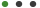Friendship Week Special | Get Additional 10% OFF, use Code "FRIENDS"
Sale Ends In

# 3 Minute Maths - FractionsLearn maths in simple, easy to understand chunks that you can build together and apply to real-life situations Kieran Ball Teaching & Academics, Math

• Introduction
1 Lectures
• Fractions
37 Lectures
• #### Description

This  is a complete set of lessons made for students of any level and any age to learn all about fractions.

I start by looking at how to find a fraction of a whole number, and  then lead onto further topics involving fractions, ending with a section  full of mixed practice questions.

Each lesson is kept short and simple, so that even the busiest student can learn. All you have to do is watch one three-minute lesson a day, and you'll be amazed at how much you pick up and remember.

The topics covered include:

• Finding fractions of whole numbers

• Subtracting fractions

• Multiplying fractions

• Dividing fractions

• Simplifying fractions

• Improper fractions

• Mixed fractions

#### What Will I Get ?

• You will learn all about fractions, and how you can use them and manipulate them
• You will learn to find fractions of whole numbers
• You will learn to add fractions, subtract them, multiply and divide them
• You will learn how to change fractions and put them into their simplest form
• You will learn about the difference between improper fractions and mixed numbers and how to change between the two
• You will also learn some hints and tips on how to recognise factors of numbers

#### Requirements

• You should be able to do basic arithmetic in order to get the most out of this course (basic adding, subtracting, multiplying and dividing)## Preview Videos

This Course Includes :

2 hours

38 Lectures